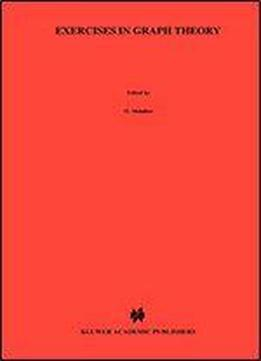# Exercises In Graph Theory (texts In The Mathematical Sciences) by O. Melnikov / 2010 / English / PDF

This book supplements the textbook of the authors" Lectures on Graph The­ ory"  by more than thousand exercises of varying complexity. The books match each other in their contents, notations, and terminology. The authors hope that both students and lecturers will find this book helpful for mastering and verifying the understanding of the peculiarities of graphs. The exercises are grouped into eleven chapters and numerous sections accord­ ing to the topics of graph theory: paths, cycles, components, subgraphs, re­ constructibility, operations on graphs, graphs and matrices, trees, independence, matchings, coverings, connectivity, matroids, planarity, Eulerian and Hamiltonian graphs, degree sequences, colorings, digraphs, hypergraphs. Each section starts with main definitions and brief theoretical discussions. They constitute a minimal background, just a reminder, for solving the exercises. the presented facts and a more extended exposition may be found in Proofs of the mentioned textbook of the authors, as well as in many other books in graph theory. Most exercises are supplied with answers and hints. In many cases complete solutions are given. At the end of the book you may find the index of terms and the glossary of notations. The "Bibliography" list refers only to the books used by the authors during the preparation of the exercisebook. Clearly, it mentions only a fraction of available books in graph theory. The invention of the authors was also driven by numerous journal articles, which are impossible to list here.

views: 177